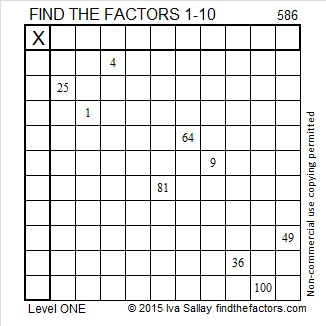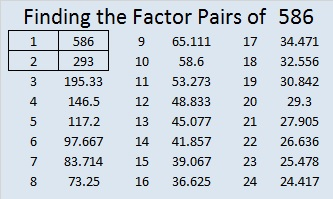# 586 and Level 1

586 is the hypotenuse of the Pythagorean triple 136-570-586. Which factor of 586 is the greatest common factor of those three numbers?Print the puzzles or type the solution on this excel file: 10 Factors 2015-08-17

—————————————————————————————————

• 586 is a composite number.
• Prime factorization: 586 = 2 x 293
• The exponents in the prime factorization are 1 and 1. Adding one to each and multiplying we get (1 + 1)(1 + 1) = 2 x 2 = 4. Therefore 586 has exactly 4 factors.
• Factors of 586: 1, 2, 293, 586
• Factor pairs: 586 = 1 x 586 or 2 x 293
• 586 has no square factors that allow its square root to be simplified. √586 ≈ 24.2074—————————————————————————————————